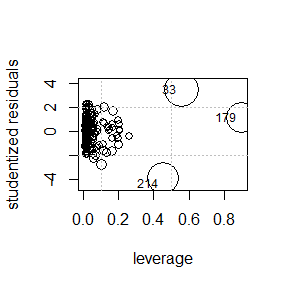# Influential Observations Diagnostics

#### 2022-09-23

An influential observation dramatically changes the fitted model based on whether it is included or excluded from the analysis.

library(api2lm)

Influential observations are often extreme with respect to their response values or their associated predictors.

• An outlier is an observation with a large residual.
• The response is relatively far from its fitted value.
• A leverage point is an observation whose predictor values are extreme relative to most other observations.

# home_sales data

To facilitate our discussion we will use the home_sales data set in the api2lm package, which contains information about homes sold in King County, WA between May 2014 and May 2015. The data set is a data frame with 216 rows and 8 columns:

• price: sale price (log10 units).
• bedrooms: number of bedrooms.
• bathrooms: number of bathrooms.
• sqft_living: size of living space.
• sqft_lot: size of property.
• floors: number of floors.
• waterfront: a factor variable with levels "yes" and "no" that indicate whether a home as a waterfront view.
• condition: a factor variable indicating the condition of a house with levels ranging from "poor" to "very good".

data(home_sales, package = "api2lm")

The validity of the assumptions we want to check depend on the model fit to the data.

We will regress the price variable on all of the remaining variables in home_sales.

lmod <- lm(price ~ ., data = home_sales)
coef(lmod)
##        (Intercept)           bedrooms          bathrooms        sqft_living
##       5.377543e+00       2.604201e-03       3.149792e-02       9.987617e-05
##           sqft_lot             floors      waterfrontyes      conditionfair
##      -8.773790e-07       8.630242e-02       3.152404e-01      -3.128967e-01
##   conditionaverage      conditiongood conditionvery_good
##      -1.278938e-01      -9.122793e-02      -2.185523e-02

# Outliers

We describe two common approaches for identifying outliers.

The simplest approach for identifying outliers is to use an index plot of the studentized residuals to determine the observations with extreme residuals.

• An index plot is a plot of a set of statistics versus their observation number.
• We will create a scatter plot of the pairs $$(i, t_i)$$ for $$i=1,2,\ldots,n$$.

The other approach is to compare the set of studentized residuals to the appropriate quantile of a $$t_{n-p-1}$$ distribution.

To evaluate whether a single observation is an outlier, we compare its studentized residual to $$t^{\alpha/2}_{n-p-1}$$, i.e., the $$1-\alpha/2$$ quantile of a $$t$$ distribution with $$n-p-1$$ degrees of freedom.

• If $$|t_i| > t^{\alpha/2}_{n-p-1}$$, then observation $$i$$ is an outlier.
• We won’t do this because we have more than one observation to evaluate.

To identify the outliers from the $$n$$ observations of our fitted model, we should adjust the previous idea using the Bonferroni correction to address the multiple comparisons problem.

• We conclude observation $$i$$ is an outlier if $$|t_i| > t^{\alpha/2n}_{n-p-1}$$.

Outlier example

The outlier_plot function is a convenient way to create an index plot of the studentized residuals from a fitted model.

outlier_plot has four main arguments that we need to know about:

• model: the fitted model.
• id_n: the number of points to identify with labels.
• add_reference: a logical value indicating whether the Bonferroni-corrected $$t$$ quantiles ($$t^{1-\alpha/2n}_{n-p-1}$$ and $$t^{\alpha/2n}_{n-p-1}$$) are added as reference lines to the plot. The default is TRUE.
• alpha: the $$\alpha$$ value used for the $$t$$ quantiles. The default is 0.05.

We use the outlier_plot function to identify the 2 most extreme outliers for our fitted model. Observations 214 and 33 have the most extreme studentized residuals and observation 214 is more extreme than the Bonferroni-adjusted $$t$$ quantile.

outlier_plot(lmod, id_n = 2)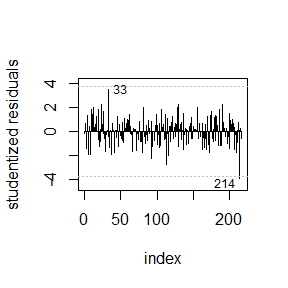The outlier_test function can be used to identify the observations with studentized residuals more extreme than the appropriate Bonferroni-corrected $$t$$ quantiles. The main arguments to the outlier_test function are:

• model: the fitted model.
• n: the number of outliers to return (by default, all of them).
• alpha: the desired familywise error rate for the family of $$n$$ tests. The default is alpha = 0.05.

Running outlier_test on a fitted model will return the identified outliers and their Bonferroni-adjusted p-values (adj_pvalue). If the adjusted p-value is less than alpha, then the observation is declared an outlier.

We use outlier_test to see that observation 214 is an outlier according to this test.

outlier_test(lmod)
##          stat       pvalue adj_pvalue
## 214 -3.948387 5.414913e-05 0.01169621

# Leverage Points

The leverage values are the diagonal elements of the hat matrix $$\mathbf{H}=\mathbf{X}(\mathbf{X}^T \mathbf{X})^{-1} \mathbf{X}^T$$. The $$i$$th leverage value is given by $$h_i=\mathbf{H}_{i,i}$$, the $$i$$th diagonal position of the hat matrix.

We can extract the leverage values of a fitted model using the hatvalues function.

An observation is declared to be a leverage point if its leverage value is unusually large.

Kutner et al. (2005) suggest two different thresholds for identifying a leverage point.

• Observation $$i$$ is declared to be an outlier if $$h_i > 2p/n$$.
• This approach can be overly sensitive.
• Observation $$i$$ is declared to be an outlier if $$h_i > 0.5$$.

Leverage example

We can create an index plot of the leverage values of a fitted model using the leverage_plot function.

The leverage_plot function takes a few main arguments:

• model: the fitted model.
• id_n: the number of points to identify with labels.
• ttype: the threshold type. The default is "half", which uses a reference threshold of 0.5. Alternatively, we can choose "2mean", which uses a reference threshold of $$2p/n$$.

We use the leverage_plot function to identify leverage points for the model fit to the home_sales data.

leverage_plot(lmod)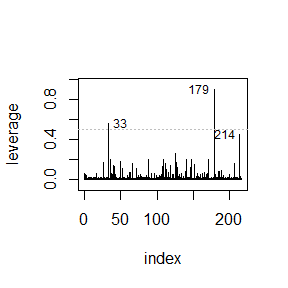Observations 33 and 179 are leverage points with leverage values greater than 0.5. Observation 214 has a leverage value nearly at 0.5, so we should consider it a leverage point.

# Influential Observations

An influential observation dramatically changes the fitted model based on whether it is included or excluded from the analysis.

We will now discuss several other measures of influence.

## DFBETA and DFBETAS

The DFBETA measure of influence is an $$n\times p$$ matrix of statistics that measures the change in the estimated regression coefficients when we fit the model using all $$n$$ observations versus when we fit the model leaving one observation out at a time.

The $$i$$th row of DFBETA is

$\text{DFBETA}_i = \hat{\boldsymbol{\beta}} - \hat{\boldsymbol{\beta}}_{(i)}.$

• $$\hat{\boldsymbol{\beta}} = [\hat{\beta}_0, \hat{\beta}_1, \ldots, \hat{\beta}_{p-1}]$$ is the vector of estimated regression coefficients when using all $$n$$ observations.
• $$\hat{\boldsymbol{\beta}}_{(i)} = [\hat{\beta}_{0(i)}, \hat{\beta}_{1(i)}, \ldots, \hat{\beta}_{p-1(i)}]$$ is the vector of estimated regression coefficients obtained from the model fit using all $$n$$ observations except the $$i$$th observation.
• $$\text{DFBETA}_{ij} = \hat{\beta}_j - \hat{\beta}_{j(i)}$$.

Identifying influential observations using the DFBETA matrix can be difficult because the sampling variance associated with each coefficient can be very different.

The DFBETAS matrix is a transformation of the DFBETA matrix that has been scaled so that the individual statistics have similar sampling variability.

DFBETAS is also an $$n\times p$$ matrix of statistics with the $$j$$th element of the $$i$$th row being defined as

$\text{DFBETAS}_{i,j} = \frac{\hat{\beta}_{j-1} - \hat{\beta}_{j-1(i)}}{\hat{\mathrm{se}}(\hat{\beta}_{j-1})},\quad i=1,2,\ldots,n, \quad j=0,1,\ldots,p-1.$

A DFBETAS statistic less than -1 or greater than 1 is often used as an indicator that the associated observation is influential in determining the fitted model.

One way of identifying influential observations is to create index plots the DFBETA or DFBETAs values for each regression coefficient and determine the estimates that substantially change when observation $$i$$ is excluded from analysis.

• We recommend using index plots of the DFFBETAS values instead of the DFBETA values because the plots will have more similar scales and are easier to work with.

The DFBETA and DFBETAS matrices can be obtained by using the dfbeta and dfbetas functions, respectively.

DFBETAS example

We can use the dfbetas_plot function to get index plots of the DFBETAS statistics for each regression coefficient.

Some of the main arguments to the dfbetas_plot function are:

• model: the fitted model.
• id_n: the number of observations for which to print an associated label.
• regressors: a formula indicating the regressors for which we want to plot the DFBETAS statistics. By default, index plots are created for all regressors.

Reference lines are automatically added at $$\pm 1$$ to indicate observations whose DFBETAS values are particularly extreme.

We plot the bedrooms, bathrooms, sqft_living, and sqft_lot variables for the fitted model of our home_sales data and label the two most extreme observations.

dfbetas_plot(lmod, id_n = 2,
regressors = ~ bedrooms + bathrooms + sqft_living + sqft_lot)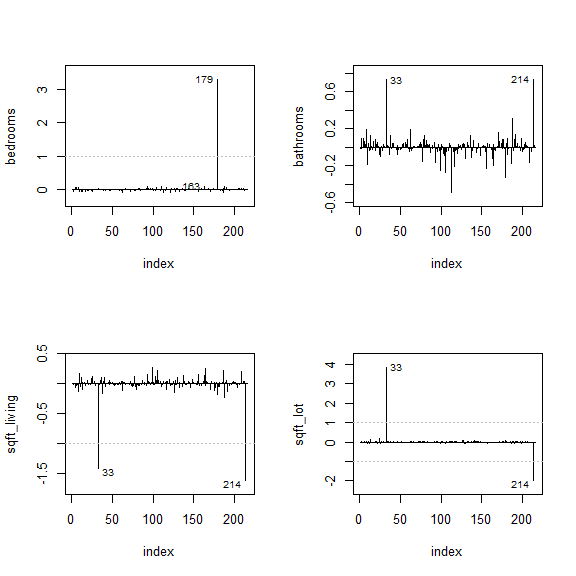We can see from these plots that:

• The estimated coefficient for bedrooms changes by more than 3 standard errors based on whether observation 179 is used in fitting the model.
• The estimated coefficients for sqft_living and sqft_lot change by more than 1 standard error based on whether observations 33 or 214 are used in fitting the model.

## DFFITS

Welsch and Kuh (1977) proposed measuring an observation’s influence through the DFFITS statistic, which is the difference between its fitted value and its LOO predicted response value.

The DFFITS statistic for observations $$i$$ defined as

$\text{DFFITS}_i = \hat{Y}_i - \hat{Y}_{i(i)}.$

• A large difference between a fitted value and its associated LOO predicted value provides evidence an observation is influential.

Belsley et al. (2005) suggest that observation $$i$$ is influential if $$|\text{DFFITS}_i|>2\sqrt{p/n}$$.

An index plot of the DFFITS statistics for all observations will indicate the observations most influential in changing their associated predicted value.

The DFFITS statistics for each observation can be obtained using the dffits function.

DFFITS example

We can create an index plot of our DFFITS statistics using the dffits_plot function.

Some of the main arguments to the dffits_plot function are:

• model: the fitted model.
• id_n: the number of observations for which to print an associated label.

Horizontal reference lines are automatically added at $$\pm 2\sqrt{p/n}$$ to identify influential observations.

We create an index plot of the DFFITS statistics below. We identify the observations with the 3 most extreme DFFITS statistics.

dffits_plot(lmod, id_n = 3)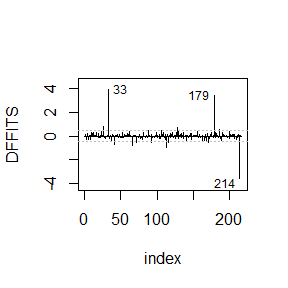We see that observations 33, 179, and 214 all have particularly extreme DFFITS statistics. Several other observations have statistics more extreme than $$\pm 1$$ (run dffits_test(lmod) for a complete list).

## Cook’s Distance

Cook (1977) proposed the Cook’s distance to summarize the potential influence of an observation with a single statistic.

The Cook’s distance for the $$i$$th observation is

$D_i=\frac{\sum_{k=1}^n (Y_k - \hat{Y}_{k(i)})^2}{p \widehat{\sigma}^2} = \frac{1}{p} r_i^2 \frac{h_i}{1-h_i}.$

• $$\hat{Y}_{k(i)}$$ is the predicted response for observation $$k$$ when observation $$i$$ is not included in the analysis (this is a type of LOO prediction).
• The standardized residual for observation $$i$$ is

$r_i = \frac{\hat{\epsilon}_i}{\widehat{\sigma}\sqrt{1-h_i}}.$

An index plot of the Cook’s distances can be used to identify observations with unusually large Cook’s distances.

• Cook’s distance values can be obtained using the cooks.distance function.
• Kutner et al. (2015) suggest declaring an observation to be influential if its Cook’s distance is more than $$F^{0.5}_{p, n-p}$$, i.e., the 0.5 quantile of an $$F$$ distribution with $$p$$ numerator degrees of freedom and $$n-p$$ denominator degrees of freedom.

Cook’s distance example

An index plot of the Cook’s distances can be created using the cooks_plot function.

Some of the main arguments to the cooks_plot function are:

• model: the fitted model.
• id_n: the number of observations for which to print an associated label.

A horizontal reference line is automatically added at $$F^{0.5}_{p,n-p}$$ to identify influential observations.

We create an index plot of the Cook’s distances below. We identify the observations with the 3 most extreme Cook’s distances.

cooks_plot(lmod, id_n = 3)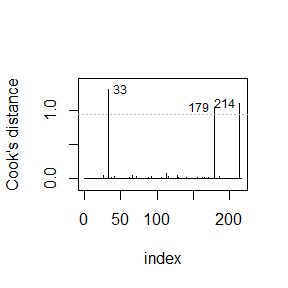We see that observations 33, 179, and 214 all have particularly extreme Cook’s distances, indicating those observations are influential in some sense. This is consistent with our previous results.

## Influence plots

An influence plot is a scatter plot of the studentized or standardized residuals versus the leverage values. The size of each point in the plot is proportional to either its Cook’s distance or DFFITS statistic.

In general, observations that are extreme with respect to their residuals, leverage values, or Cook’s distance/DFFITS statistics are more likely be be potentially influential.

Influence plot example

An influence plot can be created using the influence_plot function from the api2lm package.

Some of the main arguments to the influence_plot function are:

• model: the fitted model.
• rtype: the type of residual to plot on the y-axis. The default is the studentized residuals.
• criterion: the criterion that controls the size of the points. The default is the Cook’s distance.
• id_n: the number of observations for which to print an associated label. The most extreme observations with respect to the criterion are labeled.

We create in influence plot for the model fit to the home_sales data below.

influence_plot(lmod)